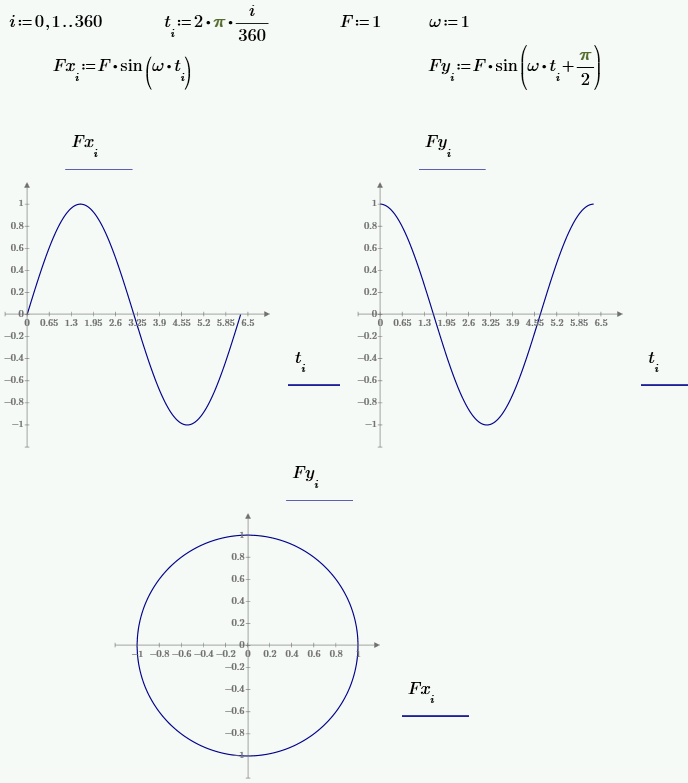peteroznewman
Subscriber
nAny force applied in a Harmonic Response analysis is entered as an amplitude of a sinusoidal function that oscillates with the frequency specified in the analysis. nHere is how you create a force that rotates around a circle... use one remote force in the X direction with an amplitude F and phase angle 0 degrees applied to a remote point. Use a second remote force in the Y direction with an amplitude F and phase angle 90 degrees applied to the same remote point. Together, those two forces create a single force of amplitude F that rotates about the origin of the remote point, parallel to the X-Y plane, with a frequency specified in the analysis.nIn the equations below, you can see a sinusoidal force in the x direction that oscillates with a circular frequency and zero phase, then another sinusoidal force in the y direction that has a pi/2 = 90 degree phase angle. When you plot the two forces in the X and Y axes, they describe a circle.n